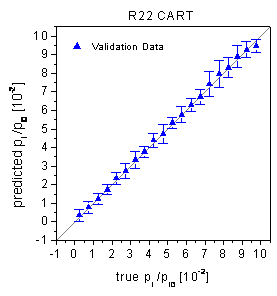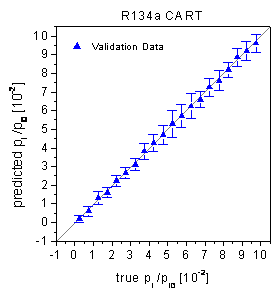Home News About Me Ph. D. Thesis Abstract Table of Contents 1. Introduction 2. Theory  Fundamentals of the Multivariate Data Analysis 3. Theory  Quantification of the Refrigerants R22 and R134a: Part I 4. Experiments, Setups and Data Sets 5. Results  Kinetic Measurements 6. Results  Multivariate Calibrations 6.1. PLS Calibration 6.2. Box-Cox Transformation + PLS 6.3. INLR 6.4. QPLS 6.5. CART 6.6. Model Trees 6.7. MARS 6.8. Neural Networks 6.9. PCA-NN 6.10. Neural Networks and Pruning 6.11. Conclusions 7. Results  Genetic Algorithm Framework 8. Results  Growing Neural Network Framework 9. Results  All Data Sets 10. Results  Various Aspects of the Frameworks and Measurements 11. Summary and Outlook 12. References 13. Acknowledgements Publications Research Tutorials Downloads and Links Contact Search Site Map Print this Page## 6.5.   CART

The classification and regression trees (CART) belong to the family of the decision trees, which are very common in the field of machine learning and medical decision support systems ,. Thereby a classification or regression problem is split into sub-problems by a binary recursive partitioning procedure. In doing so, a tree is built, which consists of nodes that assign a subpart of the tree being responsible for a specific sample and of leaves that finally assign classes or regression values to specific samples. The CART principle is an automatic machine learning method, which can deal with nonlinear classification and regression problems. The tree building process is a two-step algorithm. In the first step, the tree is built by recursively partitioning the nodes into two child nodes starting with the root node. When trying to find the maximum average "purity" of the two child nodes during a partitioning process, the CART algorithm looks for the best input variable and the corre­sponding decision criterion by a brute force approach. For the regression, the average purity is calculated by the least squares of the response variables. In easier words, the CART algorithm tries to put similar samples into the same sub-trees. This first step stops when the tree cannot grow any more resulting in an overfitting. In the second step, child nodes are pruned away, which increase the error of the calibration data less than a "size corrected" value determined in a crossvalidation procedure. Finally, for the regression the mean of the calibration samples, which are passed through the tree to a specific terminal node (leaf), is assigned to the corresponding terminal node. The prediction of a new sample is performed by passing the sample through the tree and assigning the value of the corresponding leaf.

For the calibration data set of the refrigerants, trees were built, which are shown in figure 37. For both trees, there are more leaves than concentration levels (21) of each analyte in the calibration data. This is caused by the interference of the second analyte in the mixtures. Although the CART principle is quite simple, the predictions of the calibration data are quite good with relative RMSE of 3.81% for R22 and 4.85% for R134a (see table 2). Yet, the predictions of the external validation data show the major drawback of the CART principle for the application in regressions instead of classifications with relative RMSE of 8.79% for R22 and 11.20% for R134a. The gap between the prediction of the calibration data and the validation data is enormous and dwells in the transformation of the continuous response variables into discrete variables. The experimental design used for the calibration data contains 21 different concentrations for each analyte, which are learnt as discrete values by the regression trees. The concentrations of the validation data are exactly in the middle of two neighboring concentrations of the calibration data. Consequently, the trees assign one of these two adjacent concentrations to the validation sample, which corresponds to a systematic quantization error of 5%. Thus, the gap between the validation error and the calibration error should be at least 5% for a 21-level experimental design. The rather high prediction errors of the validation data are visible in the true-predicted plots (figure 38) as rather high standard deviations. The absence of a bias in the true-predicted plots and the statistical tests for the residuals being not significant demonstrate that in principle CART is capable of accounting for the nonlinearities in the data although the quantization error renders CART impractical for this type of data.figure 37:  Decision trees built by the CART for the calibration data with green nodes and read leaves.figure 38:  True-predicted plots of the CART for the validation data.figure 39:  Relative importance of the variables for the CART predictions measured by the frequency of being used for the prediction.

In figure 39 the relative importance of the variables for the decision tree is shown. The importance reflects how often the variables are used when all samples are passed through the decision tree. The two plots show that the 2 decision trees for the 2 analytes use complemen­tary variables for the regression. The decision tree for R22 only uses variables during the sorption of analyte whereas the decision tree for R134a only uses variables during the desorption of analyte. When looking at figure 22, it is clear that the variables used for the prediction of R22 represent time points when mainly R22 has sorbed into the polymer whereas the variables used for the prediction of R134a reflect time points when all R22 has already desorbed and when practically pure R134a is left in the polymer.

The GIFI-PLS, which was proposed by Berglund et al.  for the calibration of nonlinear data, also uses a transformation of continuous response variables into discrete variables. Consequently, the GIFI-PLS approach is subject to the same quantization error and will not be investigated here any further.

 Page 94 © Frank Dieterle, 03.03.2019